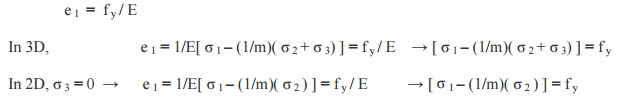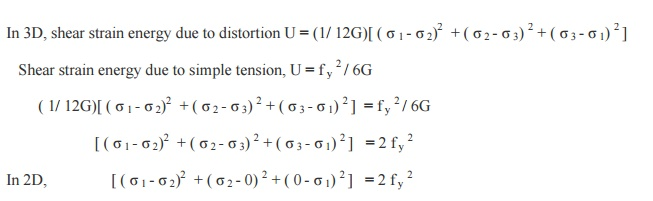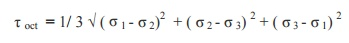Home | | Strength of Materials II | Important Questions and Answers: Strength of Materials- State of Stress in Three Dimensions

# Important Questions and Answers: Strength of Materials- State of Stress in Three Dimensions

Civil - Strength of Materials - State of Stress in Three Dimensions

STATE OF STRESS IN THREE DIMENSIONS

1. What are the types of failures?

Brittle failure:

i.Failure of a material represents direct separation of particles from each other, accompanied by considerable deformation.

ii. Ductile failure:

Slipping of particles accompanied, by considerable plastic deformations.

2.List out different theories of failure

i.                 Maximum Principal Stress Theory. ( Rakine's theory)

ii.                 Maximum Principal Strain Theory. ( St. Venant's theory)

iii.                 Maximum Shear Stress Theory. ( Tresca's theory or Guest's theory )

iv.                 Maximum Shear Strain Theory. (Von -Mises- Hencky theory or Distortion energy theory)

v.                 Maximum Strain Energy Theory. (Beltrami Theory or Haigh's theory)

3. Define: Maximum Principal Stress Theory. (Rakine's theory)

According to this theory, the failure of the material is  assumed to take place when the

value of the maximum Principal Stress (? 1) reaches a value to that of the elastic limit stress( f y) of the material. ? 1 = f y.

4. Define: Maximum Principal Strain Theory. ( St. Venant's theory)

According to this theory, the failure of the material is assumed to take place when the value of the maximum Principal Stain (e 1) reaches a value to that of the elastic limit strain( f y / E) of the material.5. Define : Maximum Shear Stress Theory. ( Tresca's theory)

According to this theory, the failure of the material is assumed to take place when the maximum shear stress equal determined from the simple tensile test.

6. Define : Maximum Shear Strain Theory (Von -Mises- Hencky theory or Distortion energy theory)

According to this theory, the failure of the material is assumed to take place when the maximum shear strain exceeds the shear strain determined from the simple tensile test.

In 3D, shear strain energy due to distortion U = (1/ 12G)[ ( ? 1 - ? 2)2  + ( ? 2 - ? 3) 2 + ( ? 3 - ? 1) 2 ]

Shear strain energy due to simple tension, U = f y 2 / 6G7. Define: Maximum Strain Energy Theory (Beltrami Theory)

According to this theory, the failure of the material is assumed to take place when the maximum strain energy exceeds the strain energy determined from the simple tensile test.8. What are the theories used for ductile failures?

1.                 Maximum Principal Strain Theory. ( St. Venant's theory)

2.                 Maximum Shear Stress Theory. ( Tresca's theory)

3.                 Maximum Shear Strain Theory. ( Von -Mises- Hencky theory or Distortion energy theory)

9. Write the limitations of Maximum Principal Stress Theory. (Rakine's theory)

1. This theory disregards the effect of other principal stresses and effect of shearing stresses on other planes through the element.

2. Material in tension test piece slips along 450 to the axis of the test piece, where normal stress is neither maximum nor minimum, but the shear stress is maximum.

3.Failure is not a brittle, but it is a cleavage failure.

10. Write the limitations of Maximum Shear Stress Theory. ( Tresca's theory).

This theory does not give the accurate results for the state of stress of pure shear in which the maximum amount of shear is developed (in torsion test).

11.Write the limitations of Maximum Shear Strain Theory.(Von -Mises- Hencky theory or Distortion energy theory).

It cannot be applied for the materials under hydrostatic pressure.

12. Write the limitations of Maximum Strain Energy Theory. ( Beltrami Theory).

This theory does not apply to brittle materials for which elastic limit in tension and in compression are quite different.

13.            Write the failure theories and its relationship between tension and shear.

1.                 Maximum Principal Stress Theory. ( Rakine's theory) ? y = f y

2.Maximum Principal Strain Theory. ( St. Venant's theory) ? y = 0.8 f y

3. Maximum Shear Stress Theory. ( Tresca's theory) ? y =0.5 f y

4.Maximum Shear Strain Theory ( Von- Mises - Hencky theory or Distortion energy theory) ? y= 0.577 f y

5.                 Maximum Strain Energy Theory. ( Beltrami Theory) ? y= 0.817f y .

14.            Write the volumetric strain per unit volume.

f y 2 / 2E

20. Define : Octahedral Stresses

A plane, which is equally inclined to the three axes of reference, is called octahedral plane. The normal and shearing stress acting on this plane are called octahedral stresses.20.            Define: Plasticity ellipse.

The graphical surface of a Maximum Shear Strain Theory (Von -Mises- Hencky theory or Distortion energy theory) is a straight circular cylinder. The equation in 2D is

? 12 -  ? 1? 2 + ? 22 = f y 2   which is called the Plasticity ellipse

Study Material, Lecturing Notes, Assignment, Reference, Wiki description explanation, brief detail
Civil : Strength of Materials : State of Stress in Three Dimensions : Important Questions and Answers: Strength of Materials- State of Stress in Three Dimensions |